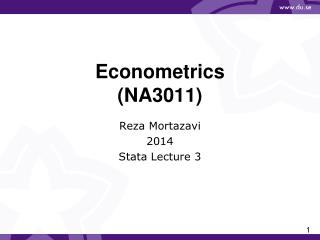Download PresentationEconometrics (NA3011)Econometrics (NA3011) - PowerPoint PPT Presentation

Download PresentationEconometrics (NA3011)
An Image/Link below is provided (as is) to download presentation

Download Policy: Content on the Website is provided to you AS IS for your information and personal use and may not be sold / licensed / shared on other websites without getting consent from its author. While downloading, if for some reason you are not able to download a presentation, the publisher may have deleted the file from their server.

- - - - - - - - - - - - - - - - - - - - - - - - - - - E N D - - - - - - - - - - - - - - - - - - - - - - - - - - -
Presentation Transcript

1. Econometrics (NA3011) Reza Mortazavi 2014 Stata Lecture 3

2. Explaining labor demand • use http://users.du.se/~rem/labour2.dta • browse • Do we have any reason to suspect heteroskedasticity? • regrlabor wage output capital • predict resid,residual

3. Testing for heteroskedasticiy • twoway (scatter resid output) • rvfplot • (Note: gives a plot of residuals against fitted values) • gen residsq=resid^2 • regrresidsq wage output capital • ereturn list • display e(N)*e(r2) • display 1- chi2(e(df_m),(e(N)*e(r2))) • (Note: chi2(df,x) is the cumulative chi-squared distribution with df degrees of freedom which gives the probability of drawing from the chi-squared distribution with df degrees of freedom and observing a value less than or equal to x.)

4. Testing for heteroskedasticiy • We don’t need to go through these steps every time we run a regression and want to test for heteroskedasticity: • regr labor wage output capital • estathettest capital output wage, iid • For our course it suffices to use: • estathettest

5. Logarithmic transformation • Let us use a log-log model: • gen lnlabor=ln(labor) • gen lnwage=ln(wage) • gen lnoutput=ln(output) • gen lncapital=ln(capital) • regrlnlaborlnwagelnoutputlncapital • How do we interpret the estimated coefficients? • Heteroskedasticity? • estat hettest • Still evidence of heteroskedastic errors

6. Robust standard errors • Regression with heteroskedasticity robust or White’s standard errors: • regress lnlaborlnwagelnoutputlncapital, vce(robust) • Compare with the previous regression. What is different? • It is recommendable that when working with cross sectional data you report these standard errors.

7. GLS • regrlnlaborlnwagelnoutputlncapital • predict ehat,residual • gen lnehatsq=ln(ehat^2) • regrlnehatsqlnwagelnoutputlncapital • Heteroskasticity? Look at the t-values for log of output and log of capital • generate hi=(exp(_b[lnwage]*lnwage+_b[lnoutput]*lnoutput+_b[lncapital]*lncapital))^0.5 • regress lnlaborlnwagelnoutputlncapital [aweight = 1/(hi^2)] • Point: given multiplicative heteroskedasticity,GLS is more efficient.